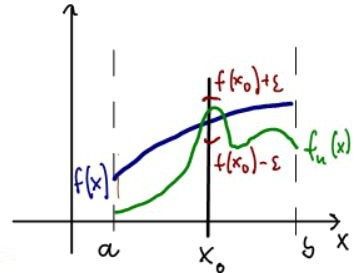#Interactive Real Analysis

Next | Previous | Glossary | Map

## 8.2. Uniform Convergence

### Definition 8.2.1: Uniform Convergence

A sequence of functions { fn(x) } with domain D converges uniformly to a function f(x) if given any> 0 there is a positive integer N such that
| fn(x) - f(x) | <for all xD whenever nN

Please note that the above inequality must hold for all x in the domain, and that the integer N depends only on.

We should compare uniform with pointwise convergence:

• For pointwise convergence we could first fix a value for x and then choose N. Consequently, N depends on bothand x.
• For uniform convergence fn(x) must be uniformly close to f(x) for all x in the domain. Thus N only depends onbut not on x.

Let's illustrate the difference between pointwise and uniform convergence graphically:

Pointwise Convergence Uniform Convergence

For pointwise convergence we first fix a value x0. Then we choose an arbitrary neighborhood around f(x0), which corresponds to a vertical interval centered at f(x0).Finally we pick N so that fn(x0) intersects the vertical line x = x0 inside the interval (f(x0) -, f(x0) +)

For uniform convergence we draw an-neighborhood around the entire limit function f, which results in an "-strip" with f(x) in the middle.Now we pick N so that fn(x) is completely inside that strip for all x in the domain.

Next | Previous | Glossary | Map